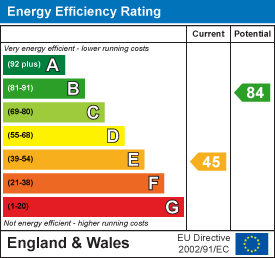•£1,425.00 To Let
•£1,425.00 To Let
•£1,425.00 To Let
•£1,425.00 To Let
•£1,425.00 To Let
•£1,425.00 To Let
•£1,425.00 To Let
•£1,425.00 To Let
•£1,425.00 To Let
•£1,425.00 To Let
•£1,425.00 To Let
•£1,425.00 To Let
•£1,425.00 To Let
•£1,425.00 To Let
•£1,425.00 To Let
•£1,425.00 To Let
•£1,425.00 To Let
•£1,425.00 To Let
•£1,425.00 To Let
•£1,425.00 To Let